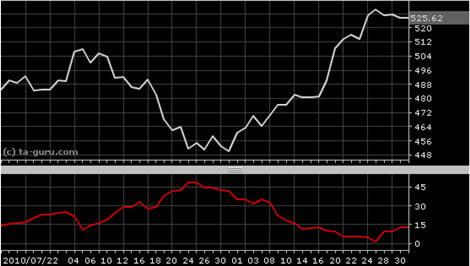Chart Pro online
User name (email):

 Recently used

 Forex

 Indices

# Negative Directional Indicator (DI-)

Negative directional indicator (DI-) is component of average directional index (ADX). If DI- is sloping forward, it is signal that downtrend is increasing. Indicator was developed by Welles Wilder.

DI- is calculated by formulas:True range (TR) indicator is used in calculation. For n parameter is usually used value of 14.Negative Directional Indicator (DI-)

Interpretation

Negative directional indicator is usually plotted with positive directional indicator (DI+). For details, see directional movement system (DMS) or average directional index (ADX).

Example